Euclideanness is quotient-closed

This article gives the statement, and possibly proof, of a commutative unital ring property (i.e., Euclidean ring) satisfying a commutative unital ring metaproperty (i.e., quotient-closed property of commutative unital rings)
View all commutative unital ring metaproperty satisfactions | View all commutative unital ring metaproperty dissatisfactions |Get help on looking up metaproperty (dis)satisfactions for commutative unital ring properties
Get more facts about Euclidean ring|Get more facts about quotient-closed property of commutative unital rings

Statement

Suppose$R$ is a commutative unital ring that possesses a Euclidean norm$N$. Suppose$I$ is an ideal inside$R$. Then,$R/I$ is a Euclidean ring, with norm given by: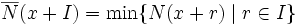$\overline{N}(x + I) = \min \{ N(x + r) \mid r \in I \}$.

In other words, the norm of a coset is defined as the minimum of normso f all elements in the coset. (Note that this minimum is well-defined since it is the minimum over a nonempty subset of a well-ordered set.

Proof

Given: A commutative unital ring$R$ with Euclidean norm$N$. An ideal$I$ of$R$.$\overline{N}$ is defined on$R/I$ by:$\overline{N}(x + I) = \min \{ N(x + r) \mid r \in I \}$.

To prove:$\overline{N}$ is a Euclidean norm on$R/I$.

Proof: Suppose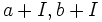$a + I, b + I$ are two elements of$R/I$ with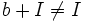$b + I \ne I$. Now, there exists$c \in a + I, d \in b + I$ with$N(c) = \overline{N}(a)$ and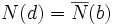$N(d) = \overline{N}(b)$. By the Euclidean division in$R$ we have: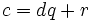$c = dq + r$

where$r = 0$ or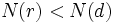$N(r) < N(d)$. Going modulo$I$, we get: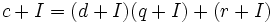$c + I = (d + I)(q + I) + (r + I)$

which can be rewritten as:$a + I = (b + I)(q + I) + (r + I)$

where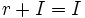$r + I = I$ or$N(r) < N(d)$. Note that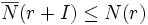$\overline{N}(r + I) \le N(r)$ by definition, and$N(d) = \overline{N}(b + I)$ by the choice of$d$. Thus, we have$r + I = I$ or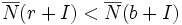$\overline{N}(r + I) < \overline{N}(b + I)$, which is precisely the condition for Euclidean division in$R/I$.